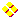WON plate26 | World!OfNumbersPalindromic Square 69696 = 2642 From “Keys to Infinity” by Clifford A. Pickover, Ed. Wiley 1995, page 159,160. 69696 is a remarkable palindrome. Not only is it a palindromic square 2642 (see also WONplate 24), not only has it exactly 10 palindromic prime factors 26 x 32 x 112, it is also the largest ' smoothly undulating ' (of the form ababab...) square known to humanity. Visit Sloane's database to find out the other smaller undulating squares at A016073. I submitted a variant namely ' undulating primes ', watch out for entry A032758.A less known fact (!?) is that 69696 is also the largest palindromic substring in  8117. 4586997231980143023221641790604173881593129978336562247475177678773845752176969616140037106220251373109248Thanks to G. L. Honaker, Jr. we know that 69696 is also the sum of a Twin Prime Pair. 69696 = 34847 + 34849[ See Sloane's A037076 and Prime Curio! 69696 ].Garland reports the following from “NUMBERS: Fun & Facts” by J. Newton Friend (1954) : 69696 is the product of two palindromes. Thus 69696 = 6336 x 11. Note 6336 is unusual in that 6336 =  8 x (63 + 36) x 8  a nice palindromic expression.[ October 24, 2005 ] Another quality of 69696 is that when it is squared and then doubled the outcome will be a pandigital number namely 9715064832 !  2 * 69696 ^ 2  Note again the palindromic expression itself ! (There exists another 5-digit palindrome with this property. Can you trace it ?)Did you know that 69696 is the product of 192 * 363 and that 192 + 363 equals 555 which is a nice repdigit ! ( Bonus : 363 – 192 = 171 yet another palindrome popping up ! )[ April 30, 2022 ] Alexandru Petrescu, from time to time obsessed with nine- and/or pandigitals, challenged himself by searching for palindromes which when multiplied with 69696 produces these nine- and pandigital numbers. He found 3 ninedigitals and 4 pandigitals. 69696 * 6996 = 487593216 69696 * 11811 = 823179456 69696 * 12821 = 893572416 69696 * 24242 = 1689570432 69696 * 42424 = 2956783104 69696 * 62526 = 4357812096 69696 * 65756 = 4582930176A000026Prime Curios!Prime PuzzleRichard PhillipsWikipedia 26Le Nombre 26Numberland 26```

```

[TOP OF PAGE]

Patrick De Geest - Belgium- Short Bio - Some Pictures